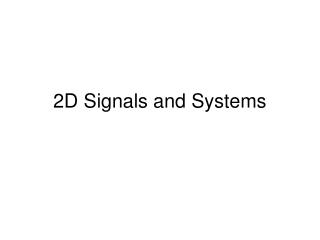Download Presentation2D Signals and Systems

# 2D Signals and Systems

Download Presentation## 2D Signals and Systems

- - - - - - - - - - - - - - - - - - - - - - - - - - - E N D - - - - - - - - - - - - - - - - - - - - - - - - - - -
##### Presentation Transcript

1. 2D Signals and Systems

2. Primitive functions in 2D h u(h,k) h (0,0) k (h,k) d d (h-1,k-1) (h,k-1) d k Plan Latex

3. 0 0 0 0 0 0 1 2 3 0 0 4 5 6 0 I(h,k) 0 7 8 9 0 0 0 0 0 0 Convolution in 2D 0 p(0,0) p(0,1) 0 0 0 0 0 p(1,0) p(1,1) 0 1 2 3 0 -1 .2 Input Image 0 4 5 6 0 I(h,k) .3 .5 Impulse Response p(h,k) 0 7 8 9 0 0 0 0 0 0 At each output Pixel: overlay, find products then add p(1,1) p(1,0) 0 Flip Impulse Response p(0,1) p(0,0) n1 = -h; n2 = -k 0 0 0 0 0 0 -1 0 0 0 g(h,k) 0 0 0 0 0 0 0

4. 0 0 0 0 0 0 1 2 3 0 0 4 5 6 0 I(h,k) 0 7 8 9 0 0 0 0 0 0 Convolution in 2D 0 p(0,0) p(0,1) 0 0 0 0 0 p(1,0) p(1,1) 0 1 2 3 0 -1 .2 Input Image 0 4 5 6 0 I(h,k) .3 .5 Impulse Response p(h,k) 0 7 8 9 0 0 0 0 0 0 At each output Pixel: overlay, find products then add p(1,1) p(1,0) 0 Flip Impulse Response p(0,1) p(0,0) n1 = -h; n2 = -k 0 0 0 0 0 0 -1 0 -.8 0 0 g(h,k) 0 0 0 0 0 0 0

5. 0 0 0 0 0 0 1 2 3 0 0 4 5 6 0 I(h,k) 0 7 8 9 0 0 0 0 0 0 Convolution in 2D 0 p(0,0) p(0,1) 0 0 0 0 0 p(1,0) p(1,1) 0 1 2 3 0 -1 .2 Input Image 0 4 5 6 0 I(h,k) .3 .5 Impulse Response p(h,k) 0 7 8 9 0 0 0 0 0 0 At each output Pixel: overlay, find products then add p(1,1) p(1,0) 0 Flip Impulse Response p(0,1) p(0,0) n1 = -h; n2 = -k 0 0 0 0 0 0 -1 0 -.8 Filter mask 0 0 g(h,k) 0 0 -3.1 0 0 0 0 0 Latex

6. Image Extension for boundary conditions Zero extension Image Periodic Extension Extension by reflection Latex

7. g(h,k) p(h,k) p(h,k) p(h,k) p(h,k) f(h,k) f(h,k) + + I(h,k) I(h,k) Convolution identities f(h,k) Associativity p(h,k) (*) g(h,k) f(h,k) = Distributivity

8. Causality (examining impulse response support) Non Causal Output Location of mask Causal Filter Support Semi-Causal Latex Or Causal if TV Raster used as causality reference

9. Filtering as a matrix operation H is Block Diagonal, Symmetric If i is extended using a periodic extension, then H becomes circulant Latex